# Group Target IX-MR Charts

Group target individual X and moving range (IX-MR) charts combine the insights of a group IX-MR chart and a target IX-MR chart to provide statistically valid information from multiple part numbers or characteristics that have a common process. Review the following example—an excerpt from Innovative Control Charting1—to get a sense of how a group target IX-MR chart works.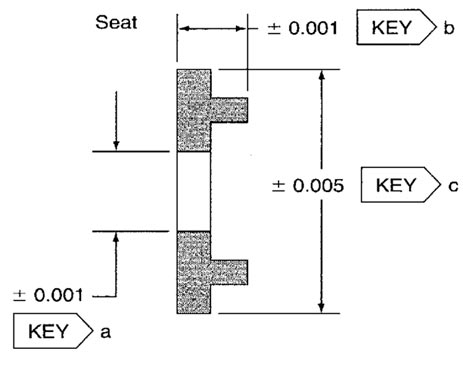Figure 1. Three generic key characteristics for the seat product line.

## Case Description

Three generic key characteristics are monitored on several different seat products. All seats share three common key characteristics and tolerances.

• Key a, inside diameter (nominal + 0.001)
• Key b, length (nominal + 0.001)
• Key c, OD (nominal + 0.005)

Seats are manufactured in many different sizes. In this example, three different seat product series (the -400, -800, and -900) will be evaluated. Each of the three seat series is machined on the same lathe but with different tools. Each characteristic is a different size, but the standard deviations are expected to be similar. The shop supervisor wants to analyze the stability of all three key characteristics, regardless of series number, on one chart (see Table 1).

Table 1. Key target values for the three different seat product series.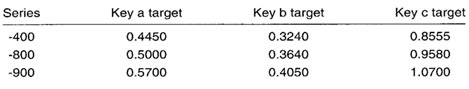## Sampling Strategy

Given low production volume and multiple characteristics of different sizes, a group target IX-MR chart is selected. This chart will help operators evaluate the variation due to the lathe and variation specific to each characteristic/product series combination. The data in Table 2 represent measurements taken at the lathe every hour in subgroup sizes of one.

## Data Collection Sheet

Table 2. Group target IX-MR data and plot points (shown in bold) for the three seat product line characteristics.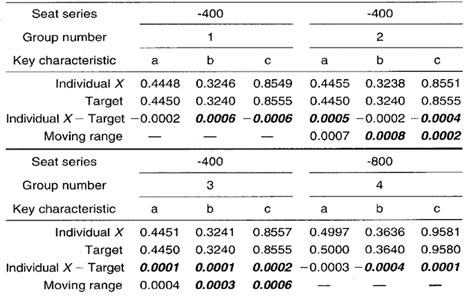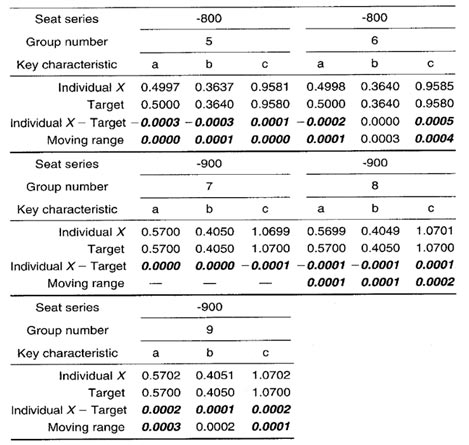## Plot Point Calculation

Group MR chart: Moving range values are calculated by taking the absolute value between individual measurements from consecutive groups for the same location. For example, location a in group 2 is 0.4455 and location a in group 1 is 0.4448, so the MR between the two groups is 10.4455 — 0.44481 = 0.0007. MAX and MIN values within each group are used as plot points.

Note: Because the same part series was not evaluated in any previous group, no MR values exist for groups 1, 4, or 7.

### Group Target IX-MR Chart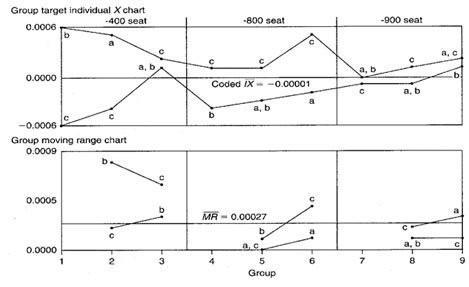Figure 2. Group target IX-MR chart used to evaluate three different key characteristics from three similar parts.

## Chart Interpretation

Note: There are only three groups per part series in this example, therefore, any plot point patterns unique to a part series should be considered only when more data become available.

Group MR chart: MAX and MIN plot points from consecutive groups appear to be descending over time. This could be the result of either

1. The standard deviation getting smaller over time regardless of part number
2. The -400 series parts exhibiting more variability than either the -800 or -900 series seats

With more data, this initial observation could be confirmed or rejected.
Note: The centerline on the group moving range chart is the average of all the moving ranges in the data set.

Group target IX chart: Key characteristic c appears in the MAX position six out of nine times. Because this is true across all three part series, it may indicate a condition inherent to the process instead of one specific to a part series. Operators speculate it has to do with the lathe’s apparent difficulty in machining the ODs. There might be something unique about why the lathe tends to run ODs a little higher than specified. Or the problem may be attributed to the programmer having written the program to intentionally manufacture the diameters on the high side. Additional investigation will be required to pinpoint the reason for this nonrandom pattern.
Note: The centerline on the group target IX chart is the average of all the coded IX plot points in the data set.

## Recommendations

• As more data are collected, the operator should pay close attention to key characteristic c (the ODs). Look for reasons why the diameters on all part series might be a little high.
• Look to see if the moving range plot points continue to decrease over time. It is possible that the -400 series key characteristics have larger standard deviations than the -800 or -900 series key characteristics. (The -800 and -900 series are larger parts, which could explain their smaller standard deviations.)

## Estimating the Process Average

If all the part series and their characteristics on the IX chart appear to be behaving randomly, a single average of all coded individual values could be used to estimate the overall process average. However, because this was not the case for the seat products here, process averages will need to be estimated for each seat characteristic across all part series. This is done by calculating a coded IX value for each characteristic for all part series. An example for characteristic a is shown in Calculation 1.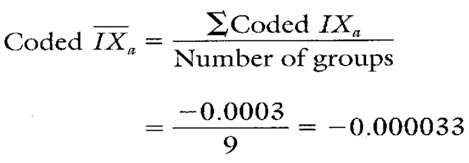Calculation 1. Estimate of the process average for key characteristic a.

## Estimating Sigma

Estimates of sigma are also calculated separately for each characteristic on the group chart. Continuing with key characteristic a, see Calculations 2 and 3.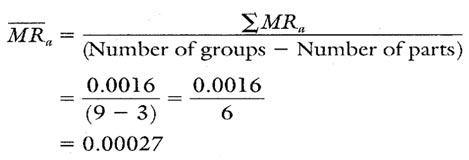Calculation 2. Calculation of MR for key characteristic a across all seat series.Calculation 3. Estimate of the process standard deviation for key characteristic a.
Note: To ensure reliable estimates, the number of groups should be at least 20. In this example, the number of groups is only 9. Therefore, these estimates and those found in Table 3 are shown only for illustration purposes.

Calculating Process Capability and Performance Ratios
These ratios are calculated using coded data. The coded target for each characteristic is zero. Calculations for key characteristic a across all three-part series are shown in Calculations 4, 5, and 6.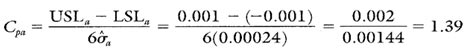Calculation 4. Cp calculation for seat key characteristic a.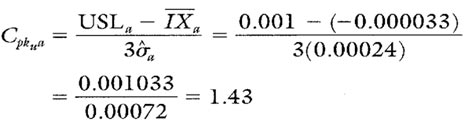Calculation 5. Cpk upper calculation for seat key characteristic a.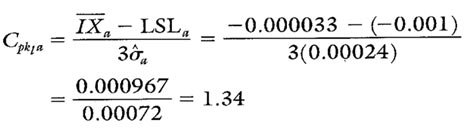Calculation 6. Cpk lower calculation for seat key characteristic a.

## Group Target IX-MR Chart Advantages

• Graphically illustrates the variation of multiple products and their characteristics simultaneously on the same chart
• Separates sources of variation unique to the process, unique to the product, and unique to a characteristic on a single chart
• Separates variation due to changes in the average from variation due to changes in the standard deviation

## Group Target IX-MR Chart Disadvantages

• No visibility of the characteristics that fall between the MAX and MIN plot points
• The use of negative numbers can be confusing
• Cannot detect certain out-of-control conditions because the group charts described here have no control limits

Additional statistics and process capability and performance values for key characteristics b and c are shown in Table 3.

Table 3. Additional statistics and process capability and performance values for key characteristics b and c.When you use SPC software from InfinityQS, consuming the information provided by group target IX-MR charts becomes faster and easier than ever. See how this type of analysis is surfaced in InfinityQS solutions.

FOOTNOTE:
1 Wise, Stephen A. and Douglas C. Fair. Innovative Control Charting: Practical SPC Solutions for Today’s Manufacturing Environment. Milwaukee, WI: ASQ Quality Press.

### Take the first step from quality to excellence

Take the Next Steps